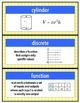# Introduction to Functions Word WallSubject
Resource Type
File Type

PDF

(13 MB|5 pages)
Product Rating
Standards
• Product Description
• StandardsNEW

This word wall includes all vocabulary to get you through an introduction to functions. There are 15 terms total:

area

circle (area formula)

cone (volume formula)

cylinder (volume formula)

discrete

function

linear equation

linear function

not discrete

rectangle (area formula)

rectangular prism (volume formula)

slope formula

sphere (volume formula)

triangle (area formula)

volume

These terms are for an INTRO to functions. They work well with the Introduction to Functions Supplementary Worksheet Pack.

*************************************************************************************************************

You might be interested in:

- these FREEBIES

- the unit on Congruence

- the unit on Linear Equations

- the unit on Percents & Proportional Relationships

or my store for other material to supplement the 7th & 8th grade curriculum!

**If you have any requests and/or updates for my work, please message me!

Interpret the equation 𝘺 = 𝘮𝘹 + 𝘣 as defining a linear function, whose graph is a straight line; give examples of functions that are not linear. For example, the function 𝘈 = 𝑠² giving the area of a square as a function of its side length is not linear because its graph contains the points (1,1), (2,4) and (3,9), which are not on a straight line.
Compare properties of two functions each represented in a different way (algebraically, graphically, numerically in tables, or by verbal descriptions). For example, given a linear function represented by a table of values and a linear function represented by an algebraic expression, determine which function has the greater rate of change.
Understand that a function is a rule that assigns to each input exactly one output. The graph of a function is the set of ordered pairs consisting of an input and the corresponding output.
Know the formulas for the volumes of cones, cylinders, and spheres and use them to solve real-world and mathematical problems.
Total Pages
5 pages
Does not apply
Teaching Duration
N/A
Report this Resource to TpT
Reported resources will be reviewed by our team. Report this resource to let us know if this resource violates TpT’s content guidelines.